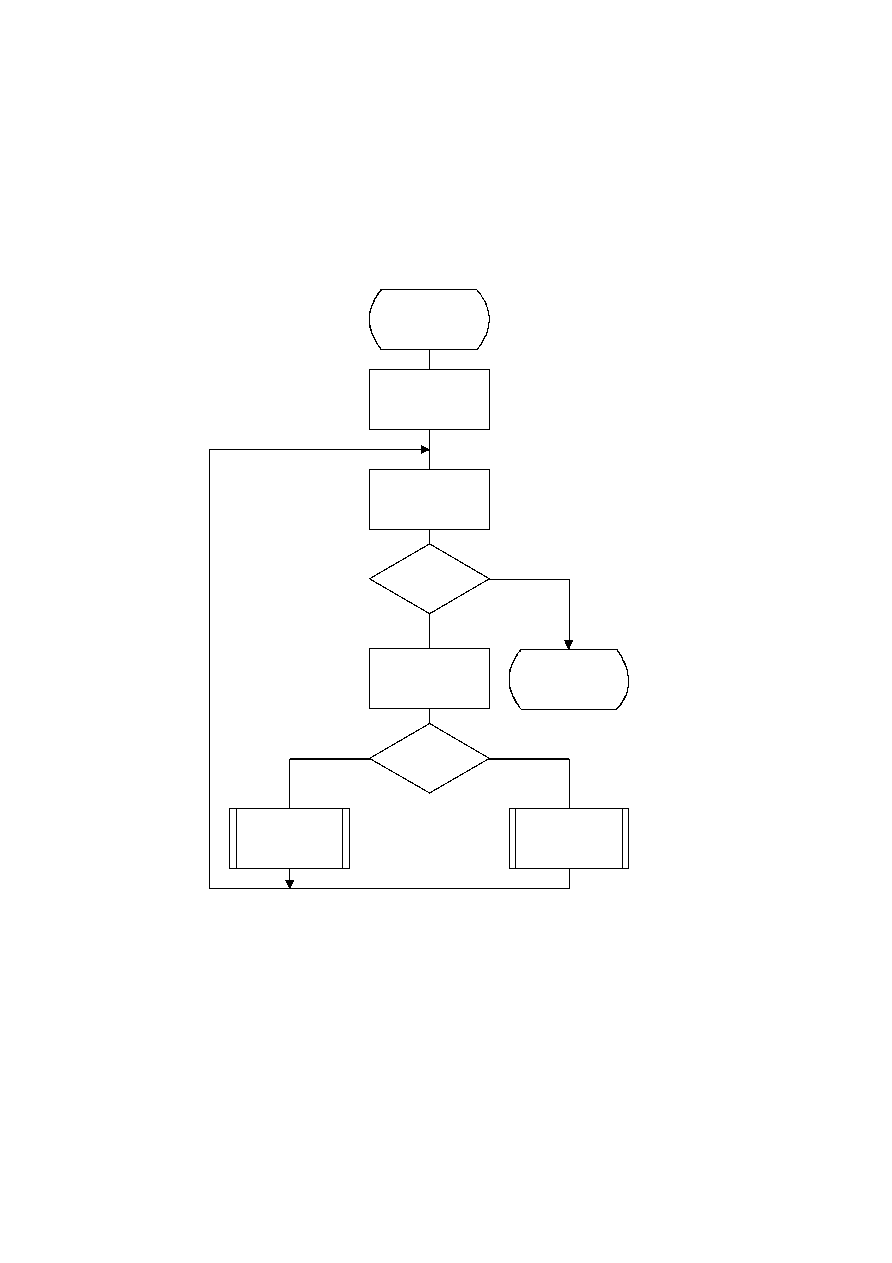ISO/IEC 10918-1 : 1993(E)
F.1.4.3.1.3
Encoding the exact value of the magnitude
After the magnitude category is encoded, the low order magnitude bits are encoded. These bits are encoded in order of
decreasing bit significance. The procedure is shown in Figure F.9. The abbreviation "SRL" indicates the shift-right-
logical operation, and M is the exclusive bound established in Figure F.8. Note that M has only one bit set ­ shifting M
right converts it into a bit mask for the logical "AND" operation.
The starting value of the context-index S is determined in Encode_log2_Sz. The increment of S by 14 at the beginning of
this procedure sets the context-index to the value required in Tables F.4 and F.5.
TISO1410-93/d079
Encode_Sz-bits
S = S + 14
M = SRL M 1
M = 0
?
Yes
No
T = M AND Sz
T = 0
?
No
Yes
Code_0(S)
Code_1(S)
Figure F.9 ­ Decision sequence to code the magnitude bit pattern
Done
Figure F.9 [D79] = 16.5 cm = 645 %
CCITT Rec. T.81 (1992 E)
99